Worksheets and No Prep Teaching Resources
Math Worksheets

# Congruent Shapes and Figures Worksheets

All congruent shape exercises are not the same, and these smart worksheets will help students understand congruence through a variety of exciting activities. Students will master congruent rectangles, squares, triangles, and angles through worksheets. More advanced options include rotation, as well as high school worksheets that cover different types of triangles and practical, customizable mixed reviews.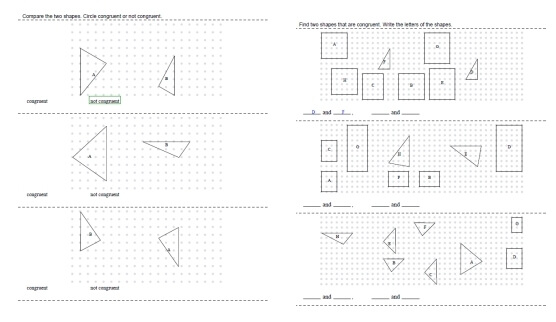Congruent or Not Congruent
Congruent Rectangles and Squares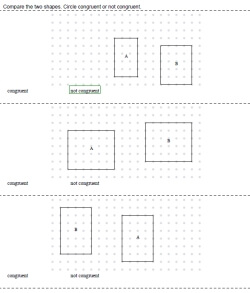Congruent Rectangles and Squares - Multiple Choice: Congruent or not congruent (first answer given) Congruent Rectangles and Squares - Multiple Choice: Circle segment, ray, line, or angle

Congruent Rectangles and Squares - More Difficult: Shapes May be Rotated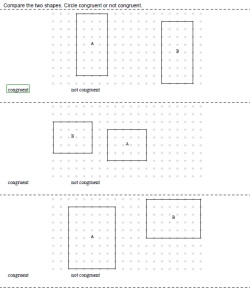Congruent Rectangles and Squares - Multiple Choice: Congruent or not congruent (first answer given) Congruent Rectangles and Squares - Multiple Choice: Circle segment, ray, line, or angle

Congruent Triangles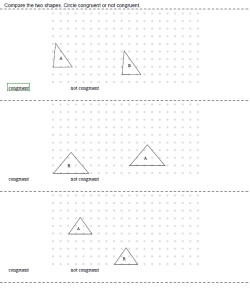Congruent Triangles - Multiple Choice: Congruent or not congruent (first answer given) Congruent Triangles - Multiple Choice: Circle segment, ray, line, or angle

Congruent Triangles - More Difficult: Shapes May be RotatedCongruent Triangles - Multiple Choice: Congruent or not congruent (first answer given) Congruent Triangles - Multiple Choice: Circle segment, ray, line, or angle

Congruent or Not Congruent - All Shapes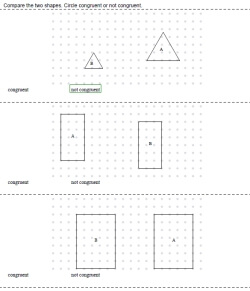Multiple Choice: Congruent or not congruent (first answer given) Multiple Choice: Circle segment, ray, line, or angle

Congruent or Not Congruent - All Shapes - More Difficult: Shapes May be Rotated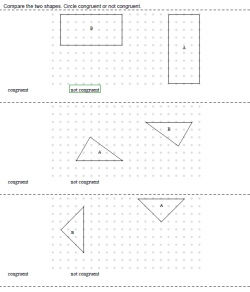Multiple Choice: Congruent or not congruent (first answer given) Multiple Choice: Circle segment, ray, line, or angle

Finding Congruent Shapes - Write All the Pairs that are Congruent
Congruent Rectangles and Squares - Easier (Shapes Are NOT Rotated)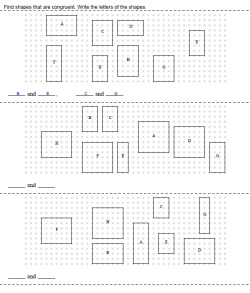Write All the Pairs that are Congruent (first pair given) Write All the Pairs that are Congruent

Congruent Rectangles and Squares - More Difficult (Shapes Are Rotated)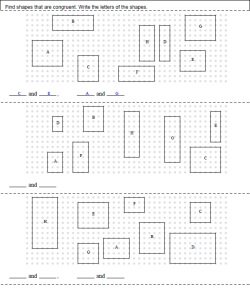Write All the Pairs that are Congruent (first pair given) Write All the Pairs that are Congruent
Congruent Triangles - Easier (Shapes Are NOT Rotated)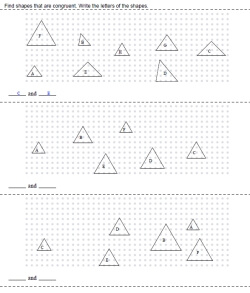Write All the Pairs that are Congruent (first pair given) Write All the Pairs that are Congruent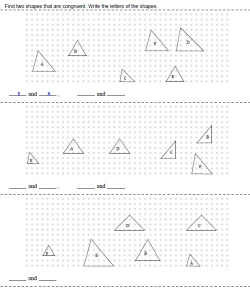Only One Pair Congruent: Write the pair that is congruent (first pair given) Only One Pair Congruent: Write the pair that is congruent

Congruent Triangles - More Difficult (Shapes Are Rotated)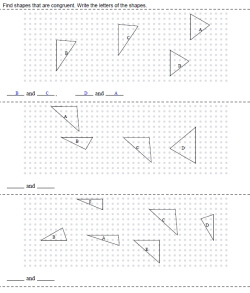Write All the Pairs that are Congruent (first pair given) Write All the Pairs that are Congruent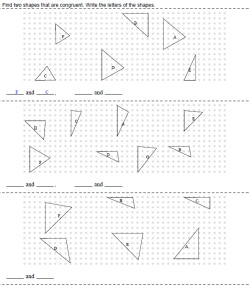Only One Pair Congruent: Write the pair that is congruent (first pair given) Only One Pair Congruent: Write the pair that is congruent
All Shapes - Easier (Shapes Are NOT Rotated)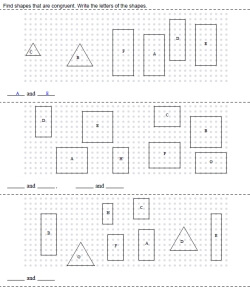Write All the Pairs that are Congruent (first pair given) Write All the Pairs that are Congruent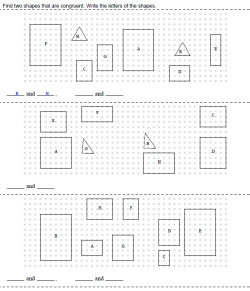Only One Pair Congruent: Write the pair that is congruent (first pair given) Only One Pair Congruent: Write the pair that is congruent

All Shapes - More Difficult (Shapes Are Rotated)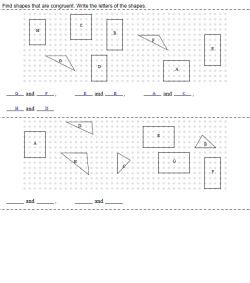Write All the Pairs that are Congruent (first pair given) Write All the Pairs that are Congruent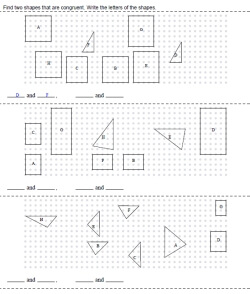Only One Pair Congruent: Write the pair that is congruent (first pair given) Only One Pair Congruent: Write the pair that is congruent

High School Congruent Printables: Congruent Triangles
Triangles and Angles
Congruence and Triangles
Proving Triangles Congruent (SAS, ASA)
Proving Triangles Congruent (AAS, SSS, HL)
Proofs using Two Sets of Triangles
Proving Triangles Congruent (Isosceles Triangles)
Congruent Triangles Mixed Review

Have a suggestion or would like to leave feedback?